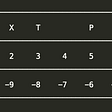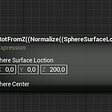# 4-digit combinations of ‘n’ numbers — using JavaScript

For numbers 0 to 9 (where n=10), the possibilities of making 4-digit combinations are 10 * 10 * 10 * 10 = 10,000.

Likewise (when n=5) any non-repeating 5 numbers totals to 5 * 5 * 5 * 5= 625 possibilities to form 4-digit combinations.

We will consider ’n’ to be ‘5’ throughout this article for convenience.

## Explanation:

The 1st digit can be chosen from (3,2,1,9,8) = 5 options

The 2nd digit can be chosen from (3,2,1,9,8) = 5 options

The 3rd digit can be chosen from (3,2,1,9,8) = 5 options

The 4th digit can be chosen from (3,2,1,9,8) = 5 options

Therefore, there are 5 * 5 * 5 * 5 (= 5⁴ = 625) possible combinations.

Similarly: For any non-repeating 5 numbers, to form 6-digit values, the total combinations are 5 * 5 * 5 * 5 * 5 * 5 (= 5⁶ = 15,625) possibilites.

In general, n pow m possibilities where m is the number of digits desired and n is the list of numbers.

When the same number is chosen more than once across one or more decimals (1’s, 10’s, 100’s, 1000's), then this condition is called as Repitition e.g. : 3333, 2298, 1339, 8998, 1288

# Possible cases:

## Case 1: Unique numbers, Repitition NOT allowed

When each number can be used only once —

example set: (3,2,1,9,8)

The 1st digit can be chosen from (3,2,1,9,8) = 5 options

The 2nd digit can be chosen from (3,2,1,9,8) minus (already chosen in step 1) = 4 options

The 3rd digit can be chosen from (3,2,1,9,8) minus (already chosen in step 1, step 2)= 3 options

The 4th digit can be chosen from (3,2,1,9,8) minus (already chosen in step 1, step2, step 3) = 2 options

Total : 5 * 4 * 3 * 2 = 120

## Case 2: Unique numbers, Repitition NOT allowed, Containing a ‘0’

If the given list of numbers consists a ’0’ & if the first digit shouldn’t be a ‘0 ’

example set: (1,0, 9,8,6)

The 1st digit can be chosen from (1,9,8,6) = 4 options

The 2nd digit can be chosen from (1,0,9,8,6) minus (already chosen in step 1) = 4 options

The 3rd digit can be chosen from (1,0,9,8,6) minus (already chosen in step 1, step 2)= 3 options

The 4th digit can be chosen from (1,0,9,8,6) minus (already chosen in step 1, step2, step 3) = 2 options

Total : 4 * 4 * 3 * 2 = 96

## Case 3: Non-Unique numbers, Repitition NOT allowed

If the given list of numbers consists one or more repeated numbers and since repitition in the final combination is NOT allowed, the total should be calculated by considering only the unique numbers.

example set: (3,1,9,8,8)

The 1st digit can be chosen from (3,1,9,8) = 4 options

The 2nd digit can be chosen from (3,1,9,8) minus (already chosen in step 1) = 3 options

The 3rd digit can be chosen from (3,1,9,8) minus (already chosen in step 1, step 2)= 2 options

The 4th digit can be chosen from (3,1,9,8) minus (already chosen in step 1, step2, step 3) = 1 option

Total : 4 * 3 * 2 * 1 = 24

## FINAL PROGRAM

Note: If the list of numbers are less than 4 (after removing duplicates), then the total count becomes ‘0’, as we cannot make even a single 4 digit number without repeating the numbers.

## BONUS

Here is a bonus function to group the list of all possibilities based on their total sum value.

Kindly let me know if the article needs correction / improvement. TIA.

--

--

--

## More from Prakash M.

learner. seeker.

Love podcasts or audiobooks? Learn on the go with our new app.

## String Indexing in Python## Getting Started on GCP: Billing Accounts## Submitting the Ambassador API Gateway to the Cloud Native Computing Foundation## Beerway Oriented Programming in F# [ 2 ]learner. seeker.

## Unleashing the full potential of Unreal Engine’s Math Expression## Let, Var and Const## Don’t mess with memset while doing CP